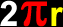# Perimeters

Formulas and instructions for finding the perimeters of circles (circumferences), kites, parallelograms, rectangles, rhombi, squares, trapezoids and oddly shaped geometric objects
Just scroll down or click on what you want and I'll scroll down for you!

 circumference of a circle perimeter of a kite perimeter of a parallelogram perimeter of a rectangle perimeter of a rhombus perimeter of a square perimeter of a trapezoid perimeter of an oddlyshaped geometric figure

 The circumference of a circle (the perimeter of a circle):The circumference of a circle is the perimeter -- the distance around the outer edge.Circumference =where r = the radius of the circleand pi = 3.141592...The perimeter of a kite: To find the perimeter of a kite, just add up all the lengths of the sides:The perimeter of a parallelogram:To find the perimeter of a parallelogram, just add up all the lengths of the sides:    Perimeter     = a + b + a + b    = 2a + 2b